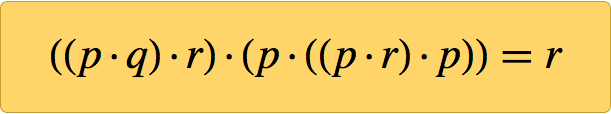# Posts from 2018

## Logic, Explainability and the Future of Understanding

For further developments, see wolframscience.com/metamathematics

## A Discovery about Basic Logic

Logic is a foundation for many things. But what are the foundations of logic itself?

In symbolic logic, one introduces symbols like p and q to stand for statements (or “propositions”) like “this is an interesting essay”. Then one has certain “rules of logic”, like that, for any p and any q, NOT (p AND q) is the same as (NOT pOR (NOT q).

But where do these “rules of logic” come from? Well, logic is a formal system. And, like Euclid’s geometry, it can be built on axioms. But what are the axioms? We might start with things like p AND q = q AND p, or  p = p. But how many axioms does one need? And how simple can they be?

It was a nagging question for a long time. But at 8:31pm on Saturday, January 29, 2000, out on my computer screen popped a single axiom. I had already shown there couldn’t be anything simpler, but I soon established that this one little axiom was enough to generate all of logic:✕ `((p·q)·r)·(p·((p·r)·p))==r`

But how did I know it was correct? Well, because I had a computer prove it. And here’s the proof, as I printed it in 4-point type in A New Kind of Science (and it’s now available in the Wolfram Data Repository):